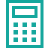### Sample Size for Comparing Means

Sample Size for Comparing Means Formula

\begin{array}{l}{n=\frac{\left(Z_{\alpha}+Z_{\beta}\right)^{2} S^{2}}{\Delta^{2}}} \\\\ {n= Sample​Size}\\\\{\text{Za}=\text { Type I error }} \\\\ {\mathrm{Z\beta}=\text { Type II error }} \\\\ {\text {S2}=\text { Historical variance }} \\\\ {\Delta 2=\text { the practical variance to be detected from the }} \\{\text { null hypothesis }}\end{array}Sample Size for Comparing Means

Invalid Input

Invalid Input

%

Invalid Input

%

Invalid Input

Invalid Input

Calculate
Thanks for using our services!
Enter some brief information to see results
• We will send you newsletters that you can opt-out for at anytime. We do not share your information.
See ResultsSample Size for Comparing MeansOne-Sided (input 1) or Two-Sided (input 2):
Probability of Stating a Difference when no Difference exists (Zα):
Probability of Stating a Difference when the Difference exists (Zβ):
Standard Deviation of Sample:
Difference to be Detected (Population Mean – Sample Mean):
Sample Size:
Reset
Example

The peel test measures the peak force required to separate a pouch seal. The mean peel strength for a new pouch seal needs to be a minimum of 4N to ensure a sterile barrier. The risk is determined to be major requiring a confidence level of 95% with a reliability of 90%. Data from the packaging qualification showed that the standard deviation was 0.5N with a mean of 4.5N. What is the sample size for the package validation study to show that the mean peel strength is a minimum of 4N?

The approach would be as follows:

• One-Sided or Two-Sided: 1
• Type I Error: 95%
• Type II Error: 90%
• Standard Deviation of Sample: 0.5
• Difference to be Detected: 0.5
• Sample Size: 9 units

A sample consisting of 9 peel strength tests would be needed to have 95% confidence that if the true mean peel strength was less than 4N it would be detected 90% of the time.

NOTES

Determining a rational sample size based on a pre-specified risk level requires the specification of the two types of risks: the risk of rejecting (e.g. package failure) when the design is actually acceptable, and the risk of accepting (e.g. package acceptance) when the design does not meet the user needs. These risks are referred to as Type I and Type II risk respectively. S2 is the historical variance. Δ is the effect size or practical difference to be detected from the null hypothesis.

The more difficult assumptions to be made are the historical variability (s) and practical difference. Historical variability can be estimated from engineering studies, similar products on the market, simulation studies or other available data. It is preferred to overestimate the variability leading to a larger sample size than underestimating the variability and not have enough power to adequately reject the null hypothesis (make a Type II error). The effect size or practical difference should reflect how much the mean value can vary from the target and still be considered acceptable to the patient/user.Sample Size for Comparing Means

Invalid Input

Invalid Input

%

Invalid Input

%

Invalid Input

Invalid Input

Calculate
Thanks for using our services!
Enter some brief information to see results
• We will send you newsletters that you can opt-out for at anytime. We do not share your information.
See ResultsSample Size for Comparing MeansOne-Sided (input 1) or Two-Sided (input 2):
Probability of Stating a Difference when no Difference exists (Zα):
Probability of Stating a Difference when the Difference exists (Zβ):
Standard Deviation of Sample:
Difference to be Detected (Population Mean – Sample Mean):
Sample Size:
Reset